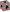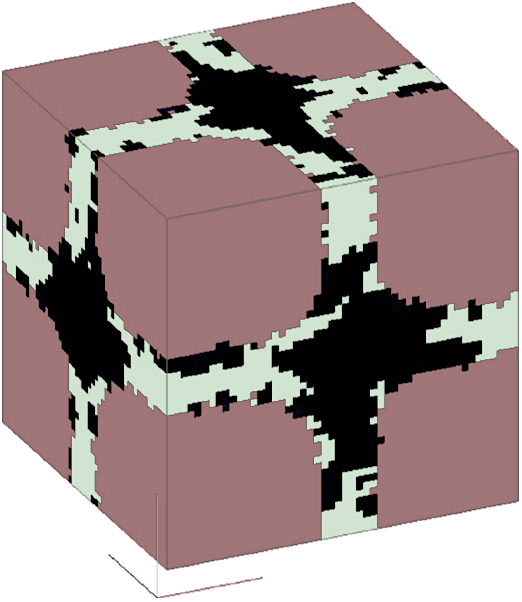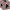# Diffusional Monte Carlo model of liquid-phase sintering

Our Monte Carlo model sheds light on the Ostwald ripening, namely the growth of big particles at the expense of the small ones.

Mathematics and Computers in Simulation 81, 2564 (2011)

A. R.S.FarrLiquid-phase sintering (LPS) is a consolidation process for metallic and ceramic powders. At given temperature conditions, the process occurs with constant amount of liquid phase. However, the evolution of solid-particle shape is observed, namely, the rounding of particles and the growth of big particles at the expense of the small ones, which is known as Ostwald ripening. In this work, we propose a Monte Carlo (MC) model to simulate the microstructural evolution during LPS. The model considers the change of state of the discretising elements, namely voxels, of the system. The microstructural evolution proceeds accounting for both the geometrical characteristics of the particles, such as the number of solid neighbours, and the amount of solute contained in or surrounding a randomly chosen voxel. This has been implemented in terms of two probability distribution functions (PDFs). The diffusion of solute has also been considered by means of the implementation of a three-dimensional finite-difference algorithm. The diffusional MC model that we present is able to reproduce the Ostwald ripening behaviour and, in particular, results match the case in which the process is limited by the diffusion of the solute in the liquid phase.#### Diffusional Monte Carlo model of liquid-phase sintering

A. R.S.Farr

Mathematics and Computers in Simulation

1 / 123 papers